# Algebra 1 : How to find mode

## Example Questions

### Example Question #1792 : Algebra 1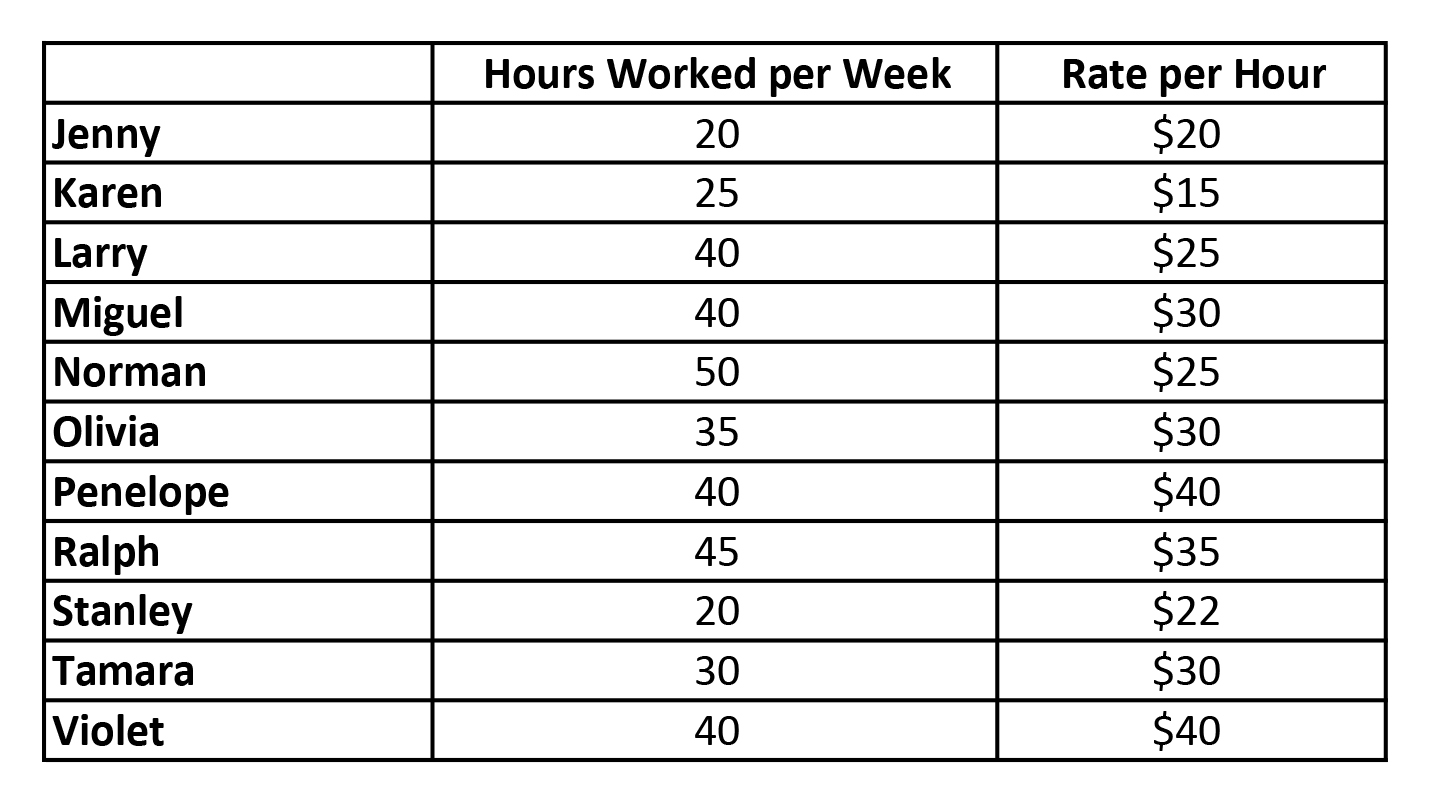Within the set of data of the hours worked per week by the staff, what is the mode?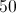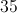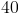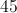Explanation:

Mode is a value that appears most often in a set of data.

In this case, 40 appears 4 times in the set of data, more than any other number.

### Example Question #2 : Mode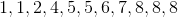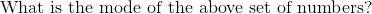Explanation: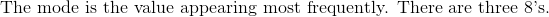### Example Question #11 : How To Find Mode

What is the mode?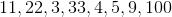NoneNone

Explanation:

Mode is the data point that occurs most frequently. In this case each data point occurs only once, so there is no mode. Answer is none.

### Example Question #12 : How To Find Mode

What is the mode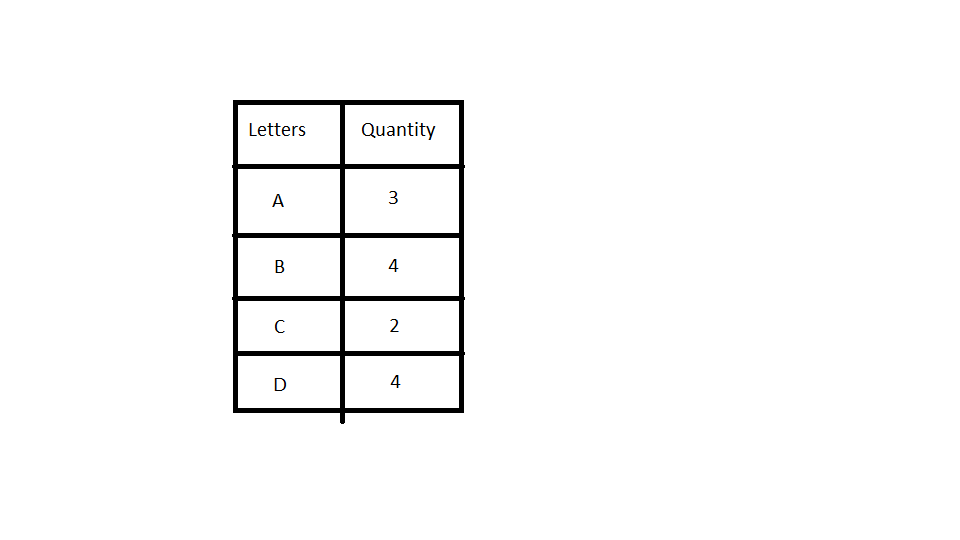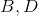NoneExplanation:

Mode is the data point that occurs most frequently. According to the data, we haveoccuring four times, followed byis three times andonly twice. The answer is just.

### Example Question #12 : How To Find Mode

What is the mode?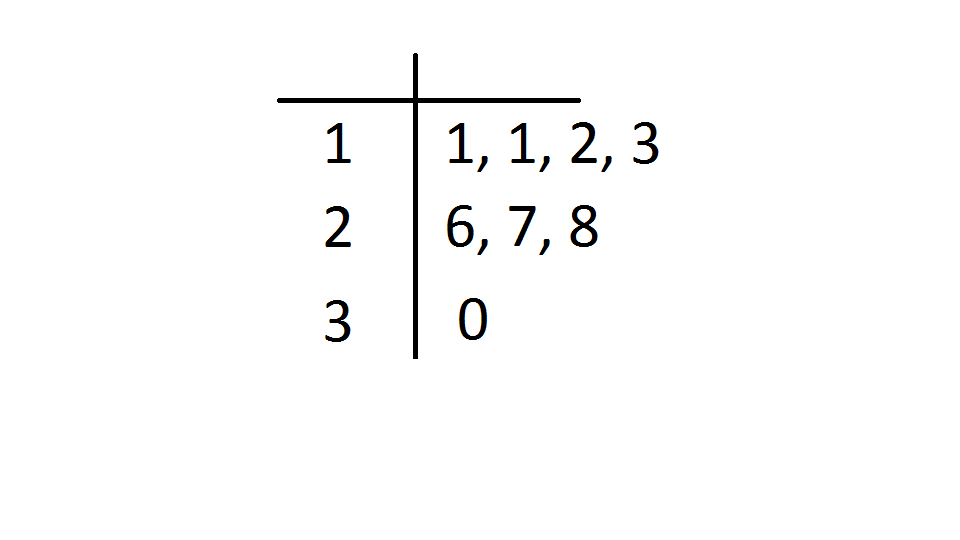NoneExplanation:

Mode is the data point that occurs most frequently. This is a stem and leaf plot where the left side represents the tens and right side is ones. The number that occurs the most iswhich is twice.

### Example Question #11 : How To Find Mode

What is the mode?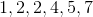Explanation:

Mode is the data point that occurs most frequently.occurs twice and is the only one that occurs more than the others.is the correct answer.

### Example Question #11 : How To Find Mode

What is the mode?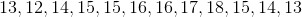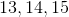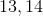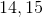Explanation:

Mode is the data point that occurs most frequently. If we arrange in increasing order, we have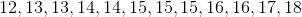occurs most frequently as it appears three times.

Some of them occur twice which isn't good enough.is the correct answer.

### Example Question #51 : Basic Statistics

What is the mode?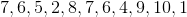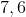Explanation:

Mode is the data point that occurs most frequently.

Let's arrange in increasing order. We have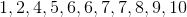andoccur twice and are the most frequent. We can have more than one mode so the answer is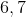### Example Question #371 : Statistics And Probability

What is the mode?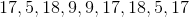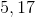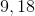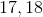Explanation:

Mode is the data point that occurs most frequently.

Let's arrange in increasing order. We have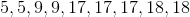occurs three times while the rest only twice.

Therefore,is the answer.

### Example Question #61 : Basic Statistics

What is the mode?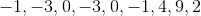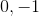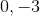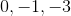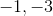Explanation:

Mode is the data point that occurs most frequently.

Let's arrange in increasing order. We have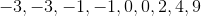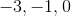occur twice while the rest only once.

Therefore,is our final answer.

### All Algebra 1 Resources